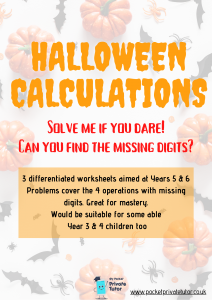Available In Stocks

Halloween themed missing number calculations using all four operations. Find the missing digits in each calculation using understanding of the formal methods. Great for mastery and proving a secure understanding of methods. Differentiated for Years 5 & 6 but could be suitable for able Year 3 and 4’s.

#### Can you find the missing digits? In each calculation, some digits have been covered over. using your understanding of how to multiply, divide, add and subtract can you find the missing digits that make each calculation correct?3 levels included:

• 1st Level is short multiplication and division with adding and subtracting 3 digit numbers and digits covered in a way that makes it easiest for children to find the first clue.
• 2nd Level is long multiplication and division with adding and subtracting 4 digit numbers and digits covered in a way that makes it easiest for children to find the first clue.
• 3rd Level is long multiplication and division with adding and subtracting 4 digit numbers and digits covered in a way that children may need to explore possibilities and use deeper reasoning skills to find each clue.

## Reviews

There are no reviews yet.